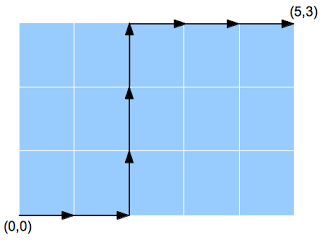## Posts

Showing posts from August, 2013

### Chess 5 Piece PuzzleSource: Mailed to me by Smriti Mittal (Final year student, IIT Bombay) Problem: Green numbers indicate how many pieces could move to that square on the next move. Blue squares show the possible locations of the following five different chess pieces: King Queen Rook Bishop Knight How are the five pieces arranged? Lets mark the squares as (r,c) where r is the row number and c is the column number. So, the squares where the pieces are to be kept are: (1,1), (1,8), (5,8), (6,3), (8,2)

### Number of Paths in Rectangular GridSource: Solved it during Algorithms Course under Prof Diwan , and discussed with Nikhil Jain (IT BHU 2008 Alumnus, Product Manager at AskLaila) Problem: 1. (Easy)  Consider a n x m rectangular grid. The problem is to find the number of shortest (or monotonic in this case) paths along the edges of the cell that start at (0,0) and end at (n,m). A monotonic path is a path always moving towards the goal, i.e. consists of moving up or right but not down or left. Figure 1 illustrates such a path for a grid of size 5 x 3. 2. (Difficult)  Now we need to print all monotonic paths that do not go above the diagonal y = x. Figure 2 shows such a path. Note that the path in figure 1 goes above the diagonal, hence not desirable in this case. How many such paths exist for n x m grid (n >= m)? Disclaimer: The first problem is too simple, and the second is very challenging. Have fun :-)

### Divisibility ProblemSource: Posted by JDGM (James Miles) in comments on http://www.puzzletweeter.com ( A fanstastic blog by Gowtham Kumar , PhD Student, Stanford, IITM Alumnus) Problem: Prove that from the numbers 1 to 200 we cannot pick 101 of them such that none divide any other. Update (24 June 2014): Solution: Posted by Suman Datta, Stainless (Ameya Ranade, CSE IITB 2009 Alumnus, Microsoft, Google, Facebook Engineer) and Wei Chen in comments!

### Most popular Puzzle Books for Technical / Quant Finance Interviews

Amazon.com Widgets

### Find Fixed Point (x[i]=i) in an Array

Source: Asked in an Amazon interview to a friend Problem: Given an array of size n , x[0 .. n-1] of integers sorted into ascending order with no duplicates, find an array item that is also its index, so that x[i] = i . For example, x = 3 in the array shown below: i           0 1 2 3 4 5 x[i]     -3 0 1 3 5 7 Your task is to write a program that finds i . Disclaimer: Yes, its a very easy problem, as far as algorithm is concerned. Its here for people preparing for interviews to see if they can write code.

### Walking Ant Problem - Part 2Source: Original problem adapted from the "Ants Problem" (Link removed) at " CMU ACM Programming Contest " . Extension to the 4 year old problem on CSE Blog - Walking Ants Puzzle  . Problem also available at  J. Paulson Programming Blog Problem: You have a bunch of ants on a meter stick, each walking 1cm/s in some direction. If an ant hits the end of the stick, it falls off. If two ants collide, they both reverse direction. Walking Ants Puzzle  earlier essentially asked: Given the starting positions and directions of all the ants, how long until the last ant falls off? The new problem is : Given the starting positions and directions of all the ants, which ant(s) are the last to fall off? Disclaimer: I do not have the solution to the problem. It just looks like an interesting problem to solve. ( Readers Please ignore: Technorati claim:  6QVZ8YSY6XSD ) Update (24 June 2014): Solution:  Posted by Strilanc and me in comments!

### Guide to Wall Street Quant Jobs for IITians

Guide to wall street quant jobs for IITians from Pratik Poddar

### Minimum Point in a Rotated Sorted Array

Source: Asked for a data scientist position at a technology startup in financial services sector in London Problem: What is the optimal algorithm to find the minimum element given a rotated sorted array of integers? A rotated sorted array of integers is the output array of a rotation operation performed on a sorted array. Eg: 3 5 6 1 2 Update (24 June 2014): Solution: Posted by Gaurav Bijay Kumar (CSE IITB 2006, Goldman Sachs Quant, Morgan Stanley Quant, Chicago Booth MBA, Credit Suisse IB, Goldman Sachs Strat), Sandeep, Khalil Sawant, Himanshu, ciddhi and gaurushh.

### Probability Puzzle: Expected Number of Expression Evaluations

Source: Asked for a data scientist position at a technology startup in financial services sector in London Problem: Given python code to calculate maximum in an array of integers x def find_max(x):          max_num = x          for i in x[1:]:         if i > max_num:             max_num = i   << Expected number of times this expression  was evaluated     return max_num Calculate the expected number of times the expression max_num = i was evaluated, given that array x was taken from a uniform random distribution.

### Combinatorics + Game Theory Puzzle

Source: Random search on http://math.stackexchange.com/ Problem: Two players A and B play the following game: Start with the set S of the first 25 natural numbers: S={1,2,…,25}. Player A first picks an even number x_0 and removes it from S: We have S:=S−{x_0}. Then they take turns (starting with B) picking a number x_n∈S which is either divisible by x_n-1 or divides x_n-1 and removing it from S. The player who can not find a number in S which is a multiple or is divisible by the previous number looses. Which player has the winning strategy and what is it?